# Solid geometry, stereometry

Solid geometry is the name for the geometry of three-dimensional Euclidean space.

Stereometry deals with the measurements of volumes of various solid figures (three-dimensional figures) including pyramids, prisms and other polyhedrons; cylinders; cones; truncated cones; and balls bounded by spheres.

1. GasholderThe gasholder has spherical shape with a diameter 20 m. How many m3 can hold in?
2. Carpenter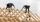From wooden block carpenter cut off a small cuboid block with half the edge length. How many percent of wood he cut off?
3. Ice cream in coneIn the ice cream cone with a diameter of 5.7 cm is 0.8 dl of ice cream. Calculate the depth of the cone.
4. Prism XThe prism with the edges of the lengths x cm, 2x cm and 3x cm has volume 20250 cm3. What is the area of surface of the prism?
5. Scale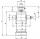Cylinder was drawn in scale 2:1. How many times is the volume of the cylinder smaller in reality?
6. Pool coating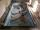How many pieces of tiles 25 cm × 15 cm need to coat the bottom and side walls of the pool with bottom dimensions 30 m × 5 m, if the pool can fit up to 271500 liters of water?
7. Air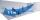Room is 35.6 m long, 19.6 dm wide and 591 cm high. How many people can simultaneously be in this room if for hygiene reasons is calculated 5000 dm3 of air per person?
8. Swimming poolThe pool shape of cuboid is 299 m3 full of water. Determine the dimensions of its bottom if water depth is 282 cm and one bottom dimension is 4.7 m greater than the second.
9. BallsThree metal balls with volumes V1=71 cm3 V2=78 cm3 and V3=64 cm3 melted into one ball. Determine it's surface area.
10. Water tank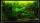Water tank shape cuboid has a width of 3.1 m and length twice larger. How high will reach water if water flow into 13 liters of water per second during 16 minutes?
11. IcerinkRectangular rink with dimensions of 68.7 m and 561 dm must be covered with a layer of ice 4.2 cm thick. How many liters of water is necessary for the formation of ice when the volume of ice is 9.7% greater than the volume of water.
12. BallsPing pong balls have a diameter of approximately 5.1 cm. They are sold in boxes of 10 pieces: each box has the shape of a cuboid with a square base. The balls touch the walls of the box. Calculate what portion of the internal volume of the box is filled
13. TanksFire tank has cuboid shape with a rectangular floor measuring 13.7 m × 9.8 m. Water depth is 2.4 m. Water was pumped from the tank into barrels with a capacity of 2.7 hl. How many barrels were used, if the water level in the tank fallen 5 cm? W
14. Building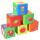Lenka has 22 cubes for the construction of building comprising three cubes in height, the width of two cubes, and the length of four cubes. Is she able to build an building with these cubes?
15. CylinderThe cylinder surface is 922 dm2, its height is equal to the radius of the base. Calculate height of this cylinder.
16. Cone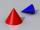If the segment of the line y = -3x +4 that lies in quadrant I is rotated about the y-axis, a cone is formed. What is the volume of the cone?
17. LatheFrom the cube of edge 37 cm was lathed maximum cylinder. What percentage of the cube is left as waste after lathed?
18. Cube zoomHow many percents do we increase the volume and surface of the cube if we magnify its edge by 38 %?
19. GutterHow much metal is needed for production 60 pieces of gutter pipes with the diameter 17 cm and length of 6 m? The plate bends add 6% of the material.
20. 2x cone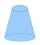Circular cone height 84 cm was cut plane parallel with base. Volume of these two small cones is the same. Calculate the height of the smaller cone.

Do you have an interesting mathematical word problem that you can't solve it? Submit math problem, and we can try to solve it.

We will send a solution to your e-mail address. Solved examples are also published here. Please enter the e-mail correctly and check whether you don't have a full mailbox.

Please do not submit problems from current active competitions such as Mathematical Olympiad, correspondence seminars etc...